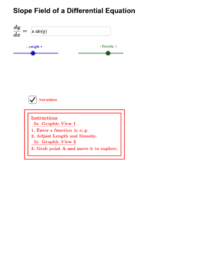# First Order DE Solving Techniques

First order differential equation is the simplest of the differential equations. In this book we put together basic techniques used for solving first order differential equations.